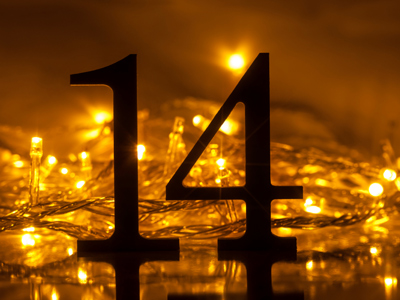Is 14 a prime number?

# Level 5-6 Algebra - Prime Numbers

You'll come across many types of numbers in KS3 Maths - integers, natural numbers, fractions, even letters which are used in place of numbers in algebra. One set of numbers you are sure to encounter is the prime numbers.

So, what exactly is a prime number? Well a prime number is an integer (whole number) with exactly two factors - no more, no less. The factors are always the number itself and the number 1. That means that 2, 3, 5, 7 and 11 are the first five prime numbers. They don't appear on any of the times tables except their own. 1 is NOT a prime number. The reason is that primes MUST have TWO factors. The number 1 has only one factor - itself.

Try this quiz all about prime numbers to see if you understand what the term really means. Read each question well and be sure to understand it before you choose your answer. And don't forget the helpful comments which come after each question. They might help to explain anything you are unsure of. Good luck!

1.
What is the next prime number after 97?
98
99
100
101
1 and 101 are the only factors of 101 and therefore it is a prime number
2.
The word 'prime' is an abbreviation for what?
Primary
Primative
Priority
It is not an abbreviation
Prime is a word in its own right
3.
Is there an easy way to work out which numbers are prime?
Yes
No
Sometimes
Only if you are a genius
Unfortunately you have to either learn the numbers or work them out
4.
What is the next prime number after 43?
44
45
46
47
You'd do well to memorise the first 30 or so prime numbers
5.
Is the number 1 a prime number?
Yes, because it is a positive whole number
No
Sometimes
Yes, because it is only divisible by itself and 1
The number 1 has only one factor - itself. Remember the rule, a prime number has TWO factors itself AND 1
6.
Which of these is a prime number?
33
35
37
39
35 appears in the 5 and the 7 times tables, 33 is in the 3 and 11 times tables and 39 (which may have tricked you) is divisible by 13 and 3
7.
Is the number 14 a prime number?
Yes
No
Yes, because it is divisible by 2
It depends which way you look at it
There are four factors of 14 - the numbers 1, 2, 7 and 14. Because there are more than two factors, it cannot be a prime number
8.
Which of these numbers is a prime number?
4
7
9
12
All the incorrect answers have three or more factors
9.
The number 24 has eight factors. Seven of the factors are 1, 2, 3, 4, 6, 12 and 24. What is the missing factor?
5
8
10
14
All the numbers given (along with the correct answer, 8) can be divided into 24 with the result that the answer is a whole number
10.
Which of these is not a prime number?
13
15
17
19
15 can be divided by 3 and 5 as well as 1 and 15
You can find more about this topic by visiting BBC Bitesize - Prime numbers, factors and multiples

Author:  Frank Evans